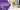# Laravel Many to Many Eloquent Relationship TutorialSumeet ShroffFebruary 10, 2023

In this article, we will be discussing the concept of many-to-many eloquent relationships in Laravel, a popular PHP framework. Laravel's Eloquent ORM provides an easy and convenient way to handle relationships between database tables. A many-to-many relationship refers to a scenario where multiple records in one table are related to multiple records in another table. This type of relationship is often implemented using a pivot table that serves as an intermediary between the two main tables. Let's consider an example to understand the concept better. Suppose you have two tables, "books" and "authors," and a book can be written by multiple authors, and an author can write multiple books. In this scenario, a many-to-many relationship exists between the "books" and "authors" tables. To implement this relationship in Laravel, we can define the relationship in the Book and Author models. The first step is to create the pivot table, which can be done using a migration in Laravel. The pivot table will contain two columns, "book_id" and "author_id," which will store the ids of the books and authors, respectively.

use Illuminate\Support\Facades\Schema;
use Illuminate\Database\Schema\Blueprint;
use Illuminate\Database\Migrations\Migration;
class CreateBookAuthorTable extends Migration
{
public function up()
{
Schema::create('book_author', function (Blueprint $table) {$table->unsignedBigInteger('book_id');
$table->unsignedBigInteger('author_id');$table->timestamps();
});
}
public function down()
{
Schema::dropIfExists('book_author');
}
}


After creating the pivot table, we can define the many-to-many relationship in the Book and Author models. In the Book model, we can use the "belongsToMany" method to define the relationship, while in the Author model, we can use the "hasMany" method.

use Illuminate\Database\Eloquent\Model;
class Book extends Model
{
public function authors()
{
return $this->belongsToMany(Author::class); } }  use Illuminate\Database\Eloquent\Model; class Author extends Model { public function books() { return$this->belongsToMany(Book::class);
}
}


Once the relationship has been defined, we can use the relationship methods to fetch the related data. For example, to get the authors of a book, we can use the following code:

$book = Book::find(1);$authors = $book->authors; Similarly, to get the books written by an author, we can use the following code: $author = Author::find(1);
$books =$author->books;

In conclusion, many-to-many relationships in Laravel can be easily implemented using the Eloquent ORM and pivot tables. With the use of relationship methods, we can fetch the related data with just a few lines of code. This makes working with relationships in Laravel a breeze and saves us a lot of time compared to writing raw SQL queries.

Laravel Many to Many Eloquent Relationship Tutorial